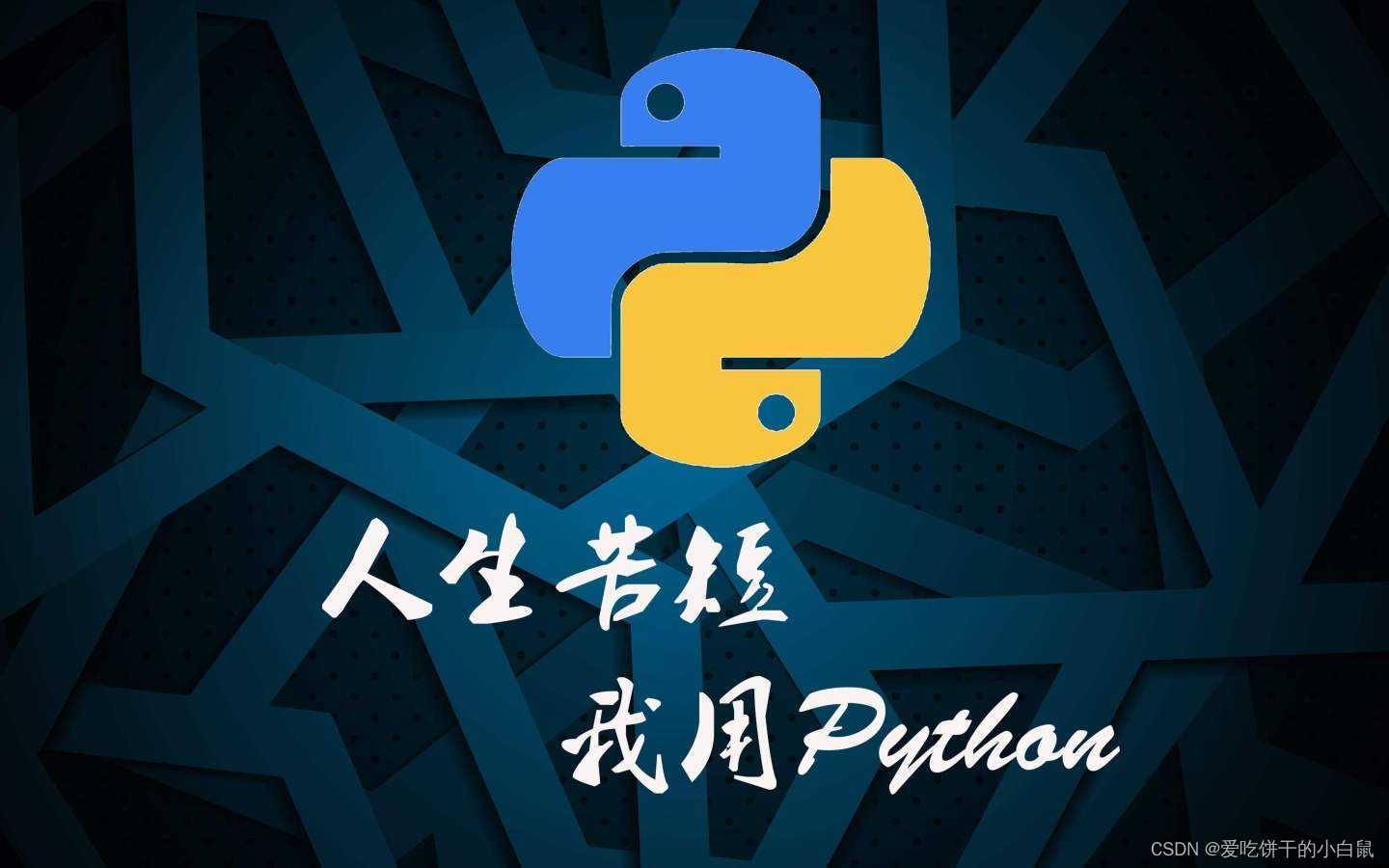# python 经典案例（3）1. 程序分析：学会分解出每一位数，如下解释.
2.程序源代码：

``````
x = int(raw_input("input a number:\n"))
a = x / 10000
b = x % 10000 / 1000
c = x % 1000 / 100
d = x % 100 / 10
e = x % 10

if a != 0:
print "there are 5 ",e,d,c,b,a
elif b != 0:
print "there are 4 ",d,c,b,a
elif c != 0:
print "there are 3 ",e,d,c
elif d != 0:
print "there are 2 ",e,d
else:
print "there are 1",e ``````

raw_input()用法

• raw_input()是Python2.X版本中的函数，在Python3.X中已经无法使用。raw_input()用于获取用户（控制台）输入，且raw_input()将所有输入都当成字符串看待。在Python3.X中input()代替了raw_input()。
• raw_input() 一般用于获取用户的字符串输入并赋值给某个变量。
• 题目：一个5位数，判断它是不是回文数。即12321是回文数，个位与万位相同，十位与千位相同。
1.程序分析：学会分解出每一位数
2.程序源代码：

``````
x = int(raw_input("input a number:\n"))
x = str(x)
for i in range(len(x)/2):
if x[i] != x[-i - 1]:
print 'this number is not a huiwen'
break
print 'this number is a huiwen'``````

题目：请输入星期几的第一个字母来判断一下是星期几，如果第一个字母一样，则继续
判断第二个字母。
1.程序分析：用情况语句比较好，如果第一个字母一样，则判断用情况语句或if语句判断第二个字母。
2.程序源代码：

• sys.stdin是一个标准化输入的方法。
• python3中sys.stdin与input的区别

• input()方法和stdin()类似，不同的是input()括号内可以直接填写说明文字。
可以看一个简单的例子：
• ``````
from sys import stdin
stdin.flush()
while letter  != 'Y':
if letter == 'S':
stdin.flush()
if letter == 'a':
print 'Saturday'
elif letter  == 'u':
print 'Sunday'
else:
print 'data error'
break
elif letter == 'F':
print 'Friday'
break
elif letter == 'M':
print 'Monday'
#break
elif letter == 'T':
stdin.flush()
if letter  == 'u':
print 'Tuesday'
elif letter  == 'h':
print 'Thursday'
else:
print 'data error'
break
elif letter == 'W':
print 'Wednesday'
else:
print 'data error'
stdin.flush()

``````

题目：求100之内的素数
1.程序分析：是指在大于1的自然数中，除了1和它本身以外不再有其他因数的自然数。
2.程序源代码：

``````
from math import sqrt
if __name__ == '__main__':
N = 100
a = range(0,N)
for i in range(2,int(sqrt(N))):
for j in range(i + 1,N):
if (a[i] != 0) and (a[j] != 0):
if a[j] % a[i] == 0:
a[j] = 0
print
for i in range(2,N):
if a[i] != 0:
print "%5d" % a[i]
if (i - 2) % 10 == 0:
print``````

Python中for in range ()是循环的次数的意思。

python中range 是个函数，range() 函数可创建一个整数列表，python中用来在for循环中遍历。

用法如： for i in range （1，3）。

语法格式：range(start, stop[, step])，分别是起始、终止和步长。

参数说明：

start: 计数从 start 开始。默认是从 0 开始。例如range(5)等价于range(0， 5);

stop: 计数到 stop 结束，但不包括 stop。例如：range(0， 5) 是[0, 1, 2, 3, 4]没有5

step：步长，默认为1。例如：range(0， 5) 等价于 range(0, 5, 1)

range函数介绍

range()函数返回的对象的行为都很像一个列表，但是它确实不是一个列表，它只是在循环迭代的情况下返回指定索引的值，但是它并不会在内存中真正产生一个列表对象，这样也是为了节约内存空间。

for i in range（）实际用法分以下几种情况：

1.for in range（3）即：从0到3，不包含3，即0,1,2。

2.for in range(1,3) 即：从1到3，不包含3，即1,2。

3.for in range（1,3,2）即：从1到3，每次增加2，因为1+2=3，不包含3，即1。第三个数字2是代表步长，如果不设置，就是默认步长为1。

物联沃分享整理
物联沃-IOTWORD物联网 » python 经典案例（3）